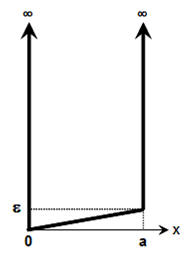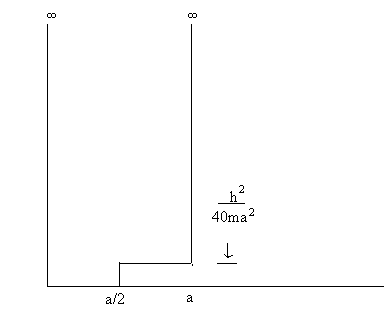### Perturbation theory, non-degenerate states

Rigid rotator

#### Problem:

The equation below defines the eigenvalue problem for a two-dimensional, quantum-mechanical, rigid rotator:
-(ħ2/2I)(∂2/∂θ2)ψ(θ) = Eψ(θ), where ψ(θ + 2π) = ψ(θ).
(a)    What are the energies Ej and wave functions ψj(θ) of its lowest three energy eigenstates?
(b)    A perturbation U = β cosθ is added to its Hamiltonian.  Find the effect on the ground state energy, to second order in β.

Solution:

• Concepts:
The rigid rotator in two dimensions, first and second order perturbation theory.
• Reasoning:
We are asked to solve the unperturbed problem and find the energy correction for the ground state due to the perturbation to second order.
• Details of the calculation:
(a)  (∂2/∂θ2)ψ(θ) + (2I/ħ2)Eψ(θ) = 0.
ψ(θ) = A exp(imθ), E = ħ2m2/(2I).
where ψ(θ + 2π) = ψ(θ) --> m = 0, ±1, ±2, ...  .
For m ≠ 0 each energy eigenvalue is two-fold degenerate.  The normalized eigenfunctions corresponding to  E = ħ2m2/(2I) are ψ±m(θ) = (2π) exp(±i|m|θ) and linear combinations.  ψ±m(θ) are eigenfunctions of Lz = (ħ/i)(∂/∂θ) with eigenvalues ±|m|ħ.

(b)  H0 + U.  U = β cosθ.
The ground state ψ0(θ) is not degenerate, E00 = 0.
E10 = <ψ0|U|ψ0> = (2π)-10 β cosθ dθ = 0.
The first order energy correction is zero.

The second order energy correction for the ground state is
E20 = ∑m≠0 |<ψm|U|ψ0>|2/(E00 - E0m).
0 exp(imθ) cosθ dθ = 0 unless |m| = 1.
E20 = |<ψ1|U|ψ0>|2/(E00 - E01) + |<ψ-1|U|ψ0>|2/(E00 - E01)
= |<ψ1|U|ψ0>|2/(-ħ2/(2I)) + |<ψ-1|U|ψ0>|2/(-ħ2/(2I))
= -(2Iβ22)2|(2π)-10 cos2θ dθ|2 = -(Iβ22).

#### Problem:

Suppose the Hamiltonian of a rigid rotator in a magnetic field is of the form
H = AL2 + BLz + CLy, if terms quadratic in the field are neglected.
Assuming B >> C, use perturbation theory to lowest non-vanishing order to get appropriate energy eigenvalues.

Solution:

• Concepts:
Stationary perturbation theory
• Reasoning:
A small correction term is added to a Hamiltonian H0, whose eigenstates and eigenvalues we can solve for exactly.  We are asked to find the lowest order non-vanishing corrections to the energy eigenvalues.
• Details of the calculation:
H = AL2 + BLz + CLy,  B >> C.
H = H0 + H', H0 =  AL2 + BLz.
The eigenstates of H0 are {|lm>}.  H0|lm> = (Al(l+1)ħ2 + Bmħ)|lm>
The eigenvalues are not degenerate.
The first order corrections are E1lm = C<lm|Ly|lm> = -(iC/2)<lm|L+- L-|lm> = 0.
We have to find the second order corrections E2lm.
E2lm = ∑l'm'≠lm C2|<l'm'|Ly|lm>|2/(E0lm - E0l'm')
Ly = -(i/2)(L+- L-)
L±|l,m> = [l(l+1) - m(m±1)]½ħ|l,m±1>.
<l'm'|L±|l,m> = [l(l+1) - m(m±1)]½ħ δl,l' δm',m±1.
E2lm = (C2/4)[l(l+1) - m(m+1)]ħ2/(Bħ(m - (m+1))) + (C2/4)[l(l+1) - m(m-1)]ħ2/(Bħ(m - (m-1)))
= +C2mħ/(2B).
The energy eigenvalues to second order are Al(l+1)ħ2 + Bmħ + C2mħ/(2B).

Infinite well

#### Problem:

A particle of mass m moves in the potential energy function

U(x) = ∞,  x < 0,  x > a,
U(x) = εx/a,  0 < x < a

where ε is a small perturbation.Use first-order perturbation theory to find the ground state energy of the particle.

Solution:

• Concepts:
First order perturbation theory for non-degenerate states
• Reasoning:
We are asked to solve the unperturbed problem and find the energy correction for the ground state due to the perturbation.
• Details of the calculation:
H = H0 + H'.  H0 is the Hamiltonian of the infinite 1D well, H' = εx/a, 0 < x < a.
The ground state eigenfunction of H0 is Φ0 = (2/a)½sin(πx/a), the associated eigenvalue is
E0 = π2ħ2/(2ma2).
The first order correction to the ground state energy is
ΔE0 = < Φ0 |H'| Φ0 > = (2/a)∫0a sin2(πx/a)(εx/a)dx = (2ε/π2) )∫0π sin2(y) y dy = ε/2.
Ground state energy to first order:  E = π2ħ2/(2ma2)  + ε/2.

#### Problem:

A particle of mass m is in an infinite potential well perturbed as shown in the figure.
(a)  Calculate the first-order energy shift of the nth eigenvalue due to the perturbation.
(b)  Write out the first three non vanishing terms for the first-order perturbation expansion of the ground state in terms of the unperturbed eigenfunctions of the infinite well.
(c)  Calculate the second-order energy shift for the ground state.Solution:

• Concepts:
First order perturbation theory for non-degenerate states
• Reasoning:
The ground state of the infinite square well is non-degenerate.
• Details of the calculation:
(a)  H = H0 + H'.
H0n> = E0nn>.   Φn(x) = (2/a)½sin(nπx/a), E0n= n2π2ħ2/(2ma2),  n = 1, 2, 3, ... .
The eigenvalues of H0 are not degenerate.
H'(x) = 0, 0 < x < a/2,  H'(x) = U0,  a/2 < x < a.  U0 = h2/(40ma2) = π2ħ2/(10ma2).
E1n = <Φn|H'|Φn> = (2U0/a)∫a/2a dx sin2(nπx/a) = U0/2.
E1n = π2ħ2/(20ma2).
To first order all energies are shifted up by U0/2.

(b)  First-order perturbation theory yields for the ket
1> = |Φ1> + ∑n>1n|H'|Φ1>/(E01 - E0n) |Φn> ,
n|H'|Φ1> = (2U0/a)∫a/2a dx sin(πx/a)sin(nπx/a)
= (2U0/π)∫π/2π dx sin(x)sin(nx)
= (2U0/π)[sin((n-1)π/2)/(2n-2) - sin((n+1)π/2)/(2n+2)].
n|H'|Φ1> = 0 if n = odd.
2|H'|Φ1> = -4U0/(3π),  <Φ4|H'|Φ1> = 8U0/(15π).
E01 - E02 = -3π2ħ2/(2ma2).  E01 - E04 = -15π2ħ2/(2ma2).
1> = |Φ1> + [4U0/(3π)]/[3π2ħ2/(2ma2)] |Φ2> - [8U0/(15π)]/[15π2ħ2/(2ma2)] |Φ4> + ...
= |Φ1> + 8/(90π) |Φ2> + 16/(2250π)|Φ4> + ... .

(c)   E21 = ∑n>1 |<Φn|H'|Φ2>|2/(E01 - E0n)
= -[4U0/(3π)]2/[3π2ħ2/(2ma2)] - [8U0/(15π)]2/[15π2ħ2/(2ma2)] - ...
= -ħ2/(100ma2)[32/27 + 128/3375 + ...] = second-order energy shift for the ground state.
The second-order energy shift of the ground state is always negative.

#### Problem:

A particle of mass m is constrained to move in an infinitely deep, one-dimensional square well extending from -a to +a.
If this particle is under the influence of a perturbation H' = -Aδ(x), where A is a constant and δ(x) is a delta function at x = 0, calculate the first order corrections to the energy levels.
What is the condition that A must satisfy if the corrected n* energy level is to have a negative energy?

Solution:

• Concepts:
First order perturbation theory for non-degenerate states, the infinite square well
• Reasoning:
The energy level of the 1D infinite square well are non-degenerate.
• Details of the calculation:
For the unperturbed Hamiltonian we have:
H = (ħ2/2m)(∂2/∂x2) + U(x),  U(x) = 0 for  |x| < a and U(x) = ∞ for  |x| > a.
(∂2/∂x2)Φ(x) + (2m/ħ2)EΦ(x) = 0 in the region |x| < a,  Φ(x) = 0 for |x| = a.
Φ(x) = Cexp(ikx) + Dexp(-ikx),   with E = ħ2k2/(2m).
Cexp(ika) + Cexp(-ika) = 0,  Cexp(-ika) + Dexp(ika) = 0.
Two solutions:
C = D: coska = 0,  ka = nπ/2, n = odd.
C = -D: sinka = 0,  ka = nπ/2, n = even.
En = n2π2ħ2/(8ma2),  Φn(x) = Ncos(nπx/(2a)), n = odd,  Φn(x) = Nsin(nπx/(2a)), n = even.
Normalization:  N2 = 1/a.

Let us calculate the first order energy corrections due to the perturbation.
n = odd:
En1 = -AN2-aa cos2(nπx/(2a))δ(x)dx = -(A/a)
n = even:
En1 = -AN2-aa sin2(nπx/(2a))δ(x)dx = 0.
En* = n2π2ħ2/(8ma2) - A/a if n = odd. We need A > n2π2ħ2/(8ma) for En* to be negative.
En* = n2π2ħ2/(8ma2) if n = even.  The perturbation does not change this energy level to first order.